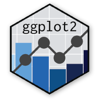Show Sidebar Hide Sidebar# interactive tooltip in ggplot2

How to add custom tooltip effects to R and ggplot2 charts with JavaScript.

### New to Plotly?

Plotly's R library is free and open source!
You can set up Plotly to work in online or offline mode.
We also have a quick-reference cheatsheet (new!) to help you get started!

### Version Check

Version 4 of Plotly's R package is now available!
Check out this post for more information on breaking changes and new features available in this version.

library(plotly)
packageVersion('plotly')

##  '4.9.1'


### Maps

library(plotly)

p <- ggplot(canada.cities, aes(long, lat)) +
coord_equal() +
geom_point(aes(text=name, size=pop), colour="red", alpha=1/2, name="cities")

p <- ggplotly(p)

p


### Custom Tooltip

library(plotly)
#install.packages("gapminder")
library(gapminder)

p <- ggplot(gapminder, aes(x = gdpPercap, y = lifeExp, color = continent, text =      paste("country:", country))) +
geom_point(alpha = (1/3)) + scale_x_log10()

p <- ggplotly(p)

p


Inspired by Gapminder Tutorial

### Control Events

library(plotly)
#install.packages("gapminder")
library(gapminder)

p <- ggplot(gapminder, aes(x = year, y = lifeExp, text = paste("country:",country))) +
geom_point() +
facet_wrap(~ continent)

p <- ggplotly(p)

p


Inspired by Gapminder Tutorial